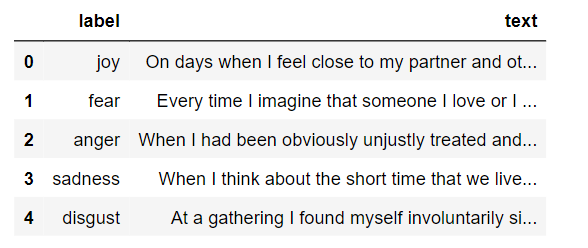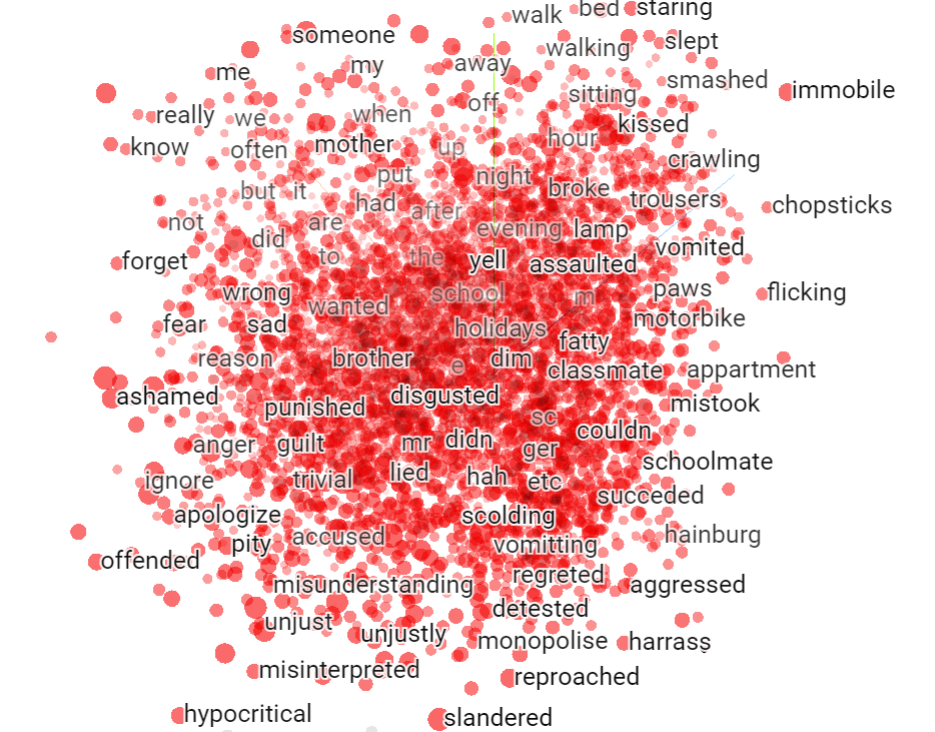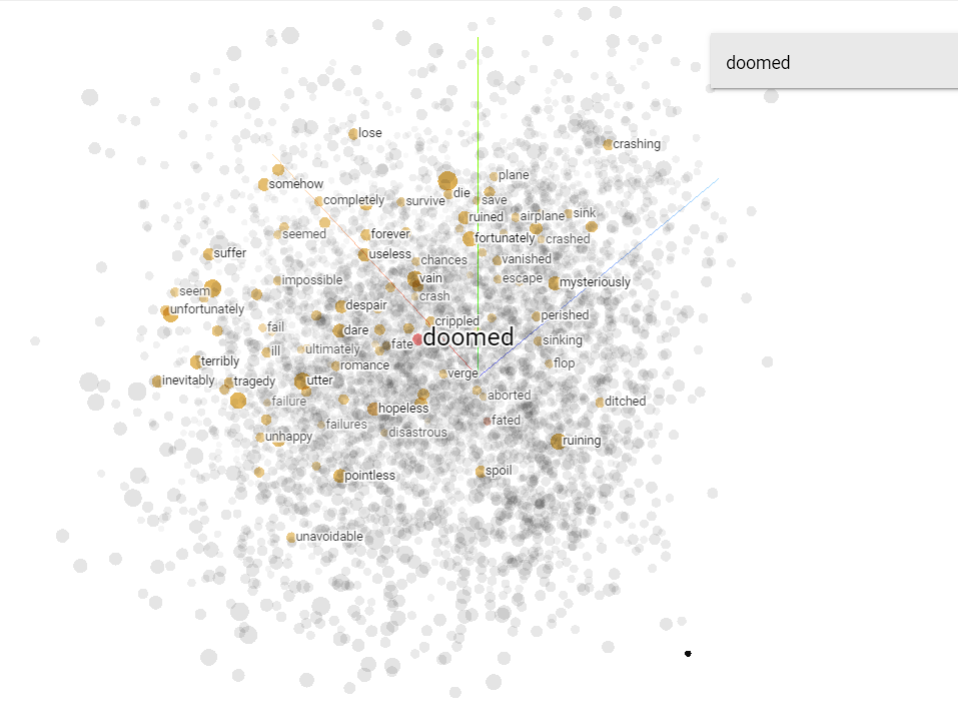Emotion Classification is a Natural Language Processing task in Machine Learning where we can process text data and classify into different classes and can detect sentiment of given text. In Natural Language Processing, we can perform different operations on text from basic text processing to classification to data extraction to text generation. With advancements in Machine Learning especially Deep Learning now we even can generate captions from images or we can also generate images from text.

So, in this example we have a dataset 7516 sentences assigned to a single class of emotion like joy, anger, fear etc. We will use deep learning to preprocess data, extract usefull information from given data and train a Deep Learning model that will classify sentences. It envolves different steps, we will use Natural Language ToolKit(NLTK) to extract keywords from sentences by removing stop words and then for Neural Network, we are using LSTM(Lont Short Term Memory). We are using Tensorflow 2 For creating Deep learning model and its layers and training of model. So, first lets import required modules and get started.

``````import pandas as pd
import numpy as np
import re
import pickle

# Import keras modules and sklearn
from keras.models import Sequential
from keras.layers.core import Activation, Dropout, Dense
from keras.layers import Flatten, LSTM, Bidirectional, Conv1D, GRU
from keras.layers.embeddings import Embedding
from sklearn.model_selection import train_test_split
from keras.preprocessing.text import Tokenizer
from sklearn.preprocessing import LabelBinarizer``````

Now, first we read csv dataset file using pandas and store as DataFrame. We can view some of the dataset examples and respective labels using DataFrame method `df.head()`.Next we need to apply some preprocessing techniques to remove unnecessary words, spaces and special characters from dataset. We can use regular experessions to search and remove a specific word or space from a sentence.

``````TAG_RE = re.compile(r'<[^>]+>')

def remove_tags(text):
return TAG_RE.sub('', text)

def preprocess_text(sen):
# Removing html tags
sentence = remove_tags(sen)

# Remove punctuations and numbers
sentence = re.sub('[^a-zA-Z]', ' ', sentence)

# Single character removal
sentence = re.sub(r"\s+[a-zA-Z]\s+", ' ', sentence)

# Removing multiple spaces
sentence = re.sub(r'\s+', ' ', sentence)

return sentence``````

To preprocess sentences, we will iterate over each sentence in dataframe and remove all html tags, extra spaces, punctuation and single characters.

``X = [preprocess_text(sen) for sent in df.text]``

Same, we need to binarize labels as currently they are in string format, so for usage we use sklearn to binarize it.

``````y = dataset['label']
# Binarize labels with SKLearn label binarizer
encoder = LabelBinarizer()
y = encoder.fit_transform(y)``````

We split dataset in two parts as training and testing dataset to get final accuracy of model after training is done.

``X_train, X_test, y_train, y_test = train_test_split(X, y, test_size=0.20, random_state=42)``

As last part of preprocessing, we need to tokenize each word and also pad it to maximum length defined.

``````# Tokenize sentencs to numbers with max number 10000
tokenizer = Tokenizer(num_words=10000)
tokenizer.fit_on_texts(X_train)

X_train = tokenizer.texts_to_sequences(X_train)
X_test = tokenizer.texts_to_sequences(X_test)

# Adding 1 because of reserved 0 index
vocab_size = len(tokenizer.word_index) + 1

maxlen = 100

Now we have training and testing data with tokenized input. We need to get embeddings for each word from embedding metrix. We will use glove embeddings for this purpose, they can be downloaded from this url.

``````# Load embedding file
embeddings_dictionary = dict()
with open("glove.6B/glove.6B.300d.txt", encoding="utf8") as glove_file:
for line in glove_file:
records = line.split()
word = records
vector_dimensions = np.asarray(records[1:], dtype='float32')
embeddings_dictionary [word] = vector_dimensions

embedding_matrix = np.zeros((vocab_size, 300))
for word, index in tokenizer.word_index.items():
embedding_vector = embeddings_dictionary.get(word)
if embedding_vector is not None:
embedding_matrix[index] = embedding_vector``````

Define a simple keras sequential model with embedding and multiple bidirectional LSTM(Long-Short term memory) layers with input if vacublary size and embedding size, output of this model will be a dense layer with softmax activation and matrix of 7 numbers as we have 7 classes in our dataset.

``````model = Sequential([
Embedding(vocab_size, 300, weights=[embedding_matrix], input_length=maxlen , trainable=False),
Bidirectional(LSTM(50, dropout=0.2, recurrent_dropout=0.2, return_sequences=True)),
Bidirectional(LSTM(54, dropout=0.3, recurrent_dropout=0.3, return_sequences=True)),
Bidirectional(LSTM(60, dropout=0.3, recurrent_dropout=0.3)),
Dense(64, activation="relu"),
Dense(7, activation="softmax")])``````

We use categorical_crossentropy for our loss function and an adam optimizer. We can view all layers and trainable parameters summary using summary function on tensorflow keras model.

``````# Compile model

# model summary
model.summary()``````

At last, we train model using training data and labels with 128 batch size. Batch size can be adjusted according to model size and GPU size to avoid OOM error. Also, we are using 20% data for validation to check model performance after each epoch. We save model stats in history variable which can later be used for checking model histroy for each epoch.

``````# Train/fit model on dataset
history = model.fit(X_train, y_train, batch_size=128, epochs=10, verbose=1, validation_split=0.2)``````

After model training is complete, we can save model, classes array and tokenizer as we will need them for inference script. This saved data can be later used for inference script and also tokens saved will help to convert new input to tokens and infer from model. We will save tensorflow model in tensorflow saved model format.

``````# Save model in tf-format
model.save("final_model")
np.save("class_names.npy", encoder.classes_) # classes can be saved in json, text or numpy format.

# Save tokenizer as pickle
with open('tokenizer.pickle', 'wb') as handle:
pickle.dump(tokenizer, handle, protocol=pickle.HIGHEST_PROTOCOL)
``````

We can also get word embeddings for each word from model weights. For this, first we get word dictionary from keras tokenizer created before.

``reverse_word_index = dict([(value, key) for (key, value) in tokenizer.word_index.items()])``

Now we get model weights data.

``````e = model.layers
weights = e.get_weights()
print(weights.shape) # shape: (vocab_size, embedding_dim)``````

Write vector files and metadata files.

``````import io

# create files for vectors and metadata
out_v = io.open('vecs.tsv', 'w', encoding='utf-8')
out_m = io.open('meta.tsv', 'w', encoding='utf-8')

# iterate over each word and get its embeddings and write in file
for word_num in range(1, vocab_size):
word = reverse_word_index[word_num]
embeddings = weights[word_num]
out_m.write(word + "\n")
out_v.write('\t'.join([str(x) for x in embeddings]) + "\n")

# close files
out_v.close()
out_m.close()``````

Tensorflow projector is an plugin for interacting with high dimensional data. We can upload our vectors and metadata to tensorflow projector and can visualize each word and their nearset words in vector space. Go to https://projector.tensorflow.org/ and click on load to upload files. Once uploaded, we can view all words in projector.Now click on a word to view its nearest points in original space.There are a lot of features in Tensorflow Projector which we can use to inspect our embeddings. For more details visit.

https://www.tensorflow.org/tensorboard/tensorboard_projector_plugin

## Inference

Now we can load our trained model, tokens and classes data and can perform inference.

``````import numpy as np
import tensorflow as tf
import pickle
import re

with open('tokenizer.pickle', 'rb') as handle:

After we have loaded our model, We can preprocess our inpiut sentence using tokenizer loaded in upper steps and convert to sequence. We also pad sentence to match max sequence length required for model.

``````sentence = 'When stole book in class and the teacher caught me the rest of the class laughed at my attempt '

``````result = model.predict(sentence_padded)